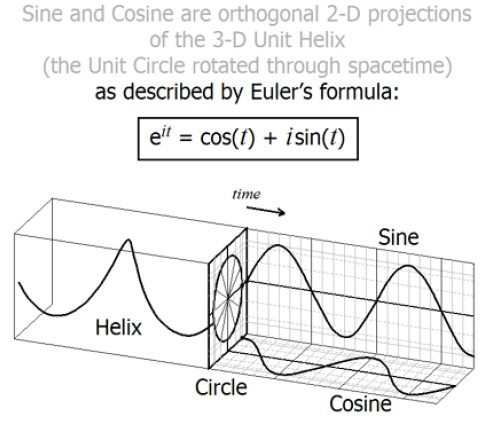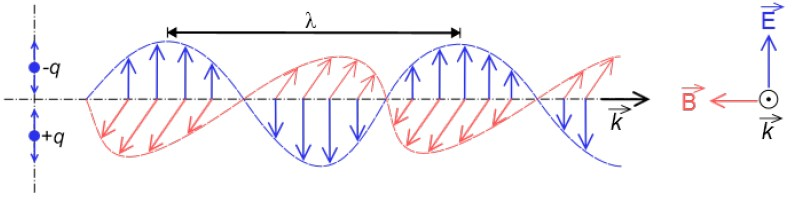# EULER’S FORMULA IS THE KEY TO UNLOCKING THE SECRETS OF QUANTUM PHYSICS

### Abstract

In this short note, the key to unlocking the secrets of quantum physics will be elucidated by exploring the fundamentals of Schrodinger’s wave mechanics approach to describing quantum phenomenon. We will show that de Broglie’s wave-particle duality hypothesis which lies at the heart of Schrodinger’s wave-function ψ produces a complex wave equation whose mathematical structure can be described by Euler’s famous equation${e}^{i\theta}=cos(\theta)+isin(\theta)$ which basically describes a helical wave in 3D space. By comparing and contrasting the electromagnetic wave with that of a helical wave which Euler’s equation represents, we may have discovered the geometric basis for spin and helicity and antimatter with negative energies that Dirac uncovered in his relativistic reformulation of Schrodinger’s equation.

Section 1: Introduction

Section 2: Schrodinger’s equation

Section 3: Euler’s formula

Section 4: Electromagnetic wave

Section 5: Dirac’s discovery and insights

Section 6: Completing the quantum revolution

Section 7: Unknown Unknowns versus Unknown Knowns

Section 8: Conclusion

References

### List of Figures

Figure 3.1. Euler’s formula and its connection to the sine and cosine waves.

Figure 4.1. This diagram shows a plane linearly-polarized EMR wave propagating from left to right

# 1 Introduction

In order to unlock the secrets of quantum physics, we have to go back in time and appreciate the challenges posed and faced by the founders of the quantum revolution. A careful reading of the ruminations of these founders as given to us in their Nobel lectures  leaves us with the feeling that something fundamental to their understanding was eluding them, as they were unable to see the key idea or concept that would have allowed them to FULLY appreciate the majesty and beauty of the quantum world. Even the last of these stalwarts Richard Feynman was dumbfounded, as reflected in his statement “I think I can safely say that nobody understands quantum mechanics”.

Using the same approach as was done in a previous note , we will go back to the basics and look at the founding assumptions and defining equations and their geometric representations, and attempt to find a pattern that lies at the heart of quantum physics by starting with the physicist who make the critical insight and the equation that was named after him. We will notice that it has a similar structure to Euler’s formula which can be represented geometrically by a helical wave in 3D space. This will then be compared to a classical electromagnetic wave in 3D space. Although both represent double harmonic oscillators, there is a distinct difference in the phase relationship that determines the physical characteristics that are found for the quantum of the field that they both represent. Finally we will discover that Dirac’s insights were already embedded in Schrodinger’s equation all along, and it was the lack of appreciation of this fundamental connection to Euler’s formula that was the stumbling block to the completion of the quantum revolution.

# 2 Schrodinger’s equation

Schrodinger’s equation  in its most general form can be represented as follows, where i is the imaginary number,$\hbar$ is Dirac’s constant or Planck’s reduced constant$\frac{h}{2\pi}$,$\frac{\partial}{\partial t}$ is the partial derivative with respect to time,$\psi$ is the wave-function, and$\hat{H}$ is the Hamiltonian operator:

(2.1)$i\hbar\frac{\partial}{\partial t}\psi=\hat{H}\psi$

This equation was based on de Broglie’s hypothesis of the wave-particle duality, in which he ascribed wave-like representations, such as angular frequency ω and wave-number k to each particle. By using the Planck-Einstein relation$E=\hbar\omega$ and de Broglie’s relation$p=\hbar k$, Schrodinger discovered a wave-function$\psi$ that codified the energy E and momentum p of the particle to these wavelike representations, where A is the wave amplitude and e is the exponential function.

(2.2)$\psi =A{e}^{i(k.r-\omega t) }=A{e}^{\frac{i(p.r-Et)}{\hbar}}$

It can be shown  that the time derivative corresponds to the energy operator$\hat { E } =i\hbar\frac{\partial}{\partial t}$, and the spatial derivative corresponds to the momentum operator$\hat{p} =i\hbar\nabla$. By finding the eigenvalues of these operators that represent the observables, one can calculate the quantized energy and momentum of different systems. In effect, the utility and power of the wave mechanics approach rests on the identification of standing/stationary waves with the eigenvalue solving tools.

# 3 Euler’s formula

Euler’s famous formula  identifies the complex phase of the exponential function with cosine and sine functions:

(3.1)${e}^{i\theta}=cos(\theta)+isin(\theta)$

By comparing (2.2) with (3.1), and identifying with the wave-function$\psi=A{e}^{i\theta}$, then the complex phase factor$\theta=k.r-\omega t.$

An excellent insightful graphical deconstruction of Euler’s formula  shows that 1) it represent a unit circle rotated through space-time, 2) the sine wave is the orthogonal projection of the rotated unit circle, 3) cosine is also an orthogonal projection of the rotated unit circle, just 90 degrees out of phase, and 4) sine and cosine are orthogonal 2-D projections of the 3-D unit helix as summarised in Figure 3.1.

#### Figure 3.1. Euler’s formula and its connection to the sine and cosine waves. Adapted from .# 4 Electromagnetic wave

Let us now compare the 3D representation of the Euler’s wave with the traditional electromagnetic wave.

Electromagnetic waves  can be represented as a self-propagating transverse oscillating wave of electric and magnetic fields as shown in Figure 4.1. The two types of fields in the electromagnetic waves are always in phase with each other.

#### Figure 4.1. This diagram shows a plane linearly-polarized EMR wave propagating from left to right. The electric field is in a vertical plane and the magnetic field in a horizontal plane. Adapted from .There are many similarities between both waves. Both consist of alternating sinusoidal waves in planes orthogonal to each other and to the direction of propagation, with the major and crucial difference being that the sinusoidal waves are symmetrical (sine/sine) and in phase in the case of the electromagnetic waves; whereas they are anti-symmetrical (sine/cosine) and out of phase by 90 degrees in the case of the Euler’s waves.

# 5 Dirac’s discovery and insights

Dirac discovered from his relativistic version of the wave mechanics approach that the electron wave has$\frac{1}{2}$ spin and helicity and also that it predicts the existence of antimatter with negative energies.  Is it possible that the insights gained from his algebraic approach can be understood geometrically from the fact that the sine and cosine waves are out of phase by 90 degrees, and also from the fact that the square of isin(θ) is always negative, respectively?

# 6 Completing the quantum revolution

The lessons that have been learnt thus far is that unlike the conventional picture that space-time is continuous and matter is discrete, the converse may be closer to the truth, in which space-time is in fact discrete , while our matter waves may be continuous entities; hence helping to explain some of the mysteries of quantum mechanics such as non-locality and entanglement. We may also be closer to understanding why there are many mathematical representations of quantum physics from matrix mechanics, to wave mechanics and finally to the path integral approach. In each of these approaches, the observables are described by the stationary/standing waves states where the solutions are basically the eigenvalues, which ultimately follow from the fundamentals of harmonic analysis .

# 7 Unknown Unknowns versus Unknown Knowns

United States Secretary of Defence Donald Rumsfeld once said , “There are known knowns; there are things we know we know. We also know there are known unknowns; that is to say we know there are some things we do not know. But there are also unknown unknowns – there are things we do not know we don’t know.” Although many of us feel that when it comes to grasping the physical reality of quantum physics, we are dealing with the unknown unknowns, I beg to differ. I feel that we are dealing with unknown knowns; there are some things we don’t know that we know. In this case, it is a matter of connecting the dots of the things that are known in order to make known “the pattern that connects” .

# 8 Conclusion

In this short note, it is hoped that I have succeeded in this regard by showing how Euler’s formula provides the machinery that unites the mathematics of the geometry, algebra and trigonometry  and provides a stepping stone or key to helping us understand the workings of our physical world. Let us now see how far we can go in using the insights of the atoms of space model provided in  and the insights derived here from the properties of Euler’s formula to constructing a complete and consistent theory of causation and fundamental interactions.

# References

 Gao, S., 2012. Six Nobel Lectures on Quantum Physics 1st ed., Amazon Kindle Direct Publishing.

 Feynman, R., 1964. The Character of Physical Law, Modern Library.

 Sahely, B., 2012.The Atoms of Space., originally posted here:  http://vixra.org/abs/1109.0030

 Schrodinger’s equation. http://en.wikipedia.org/wiki/Schr%C3%B6dinger equation

 Euler’s formula. http://en.wikipedia.org/wiki/Euler%27s formula

 This is a gif animation of about a minute duration. http://en.wikipedia.org/wiki/File:Sine and Cosine fundamental relationship to Circle %28and Helix%29.gif

 Harmonic analysis. http://en.wikipedia.org/wiki/Harmonic analysis

 Hoffmeyer,  J. ed.,  2010. A Legacy for Living Systems:  Gregory Bateson as Precursor to Biosemiotics Softcover reprint of hardcover 1st ed. 2008., Springer.

## 2 thoughts on “EULER’S FORMULA IS THE KEY TO UNLOCKING THE SECRETS OF QUANTUM PHYSICS”

1.PuMa Tse says:

Your basic premise is correct. The fundamental formula of Thermodynamics and geodesic EFE work together. The conditions shift from one to the next and inversely with the other condition as interacting circular functions. The total transformation being a quantum cycle=helix and divisible into the linear passage of time. At the extremes are mathematical singularities wherein instead of two things interacting one is interacting with itself in two simultaneous ways. Note I said mathematical.

Einstein didn’t like the mathematical definition which works for QM better than his preferred classical thinking. You can say he threw out the new thinking definitions to resort to the old thinking that created the problem. Irony! T(mu)(nu) interacts with itself as surface tension. Following the mathematical defintions inconveniently throws out a few things like wormholes, but invites more profound quantum things.

Definitely a quantum universe, and even more ambiguous than this when you step down in its architecture to our familiar and functional temporal realm. I’m still trying to find a nice way to present the whole picture in a way a normal human can comprehend. See my page, and by all means jump in (https://www.facebook.com/QuantumRelativity.PT/).

This site uses Akismet to reduce spam. Learn how your comment data is processed.